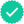Question

If f is an even function, determine whether g is even, odd, or neither. Explain.
(a)
g(x) = −f(x)
even
odd
neither

Explain.
The graph is a reflection in the x-axis.
The graph is a reflection in the y-axis.
The graph is a vertical translation of f.
The graph is a horizontal translation of f.
The graph is symmetric about the origin.

(b)
g(x) = f(−x)
even
odd
neither

Explain.
The graph is a reflection in the x-axis.
The graph is a reflection in the y-axis.
The graph is a vertical translation of f.
The graph is a horizontal translation of f.
The graph is symmetric about the origin.

(c)
g(x) = f(x) − 3
even
odd
neither

Explain.
The graph is a reflection in the x-axis.
The graph is a reflection in the y-axis.
The graph is a vertical translation of f.
The graph is a horizontal translation of f.
The graph is symmetric about the origin.

(d)
g(x) = f(x − 3)
even
odd
neither

Explain.
The graph is a reflection in the x-axis.
The graph is a reflection in the y-axis.
The graph is a vertical translation of f.
The graph is a horizontal translation of f.
The graph is symmetric about the origin.

Expert SolutionKnowledge Booster••